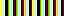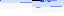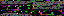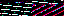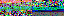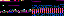```from esp_hadouken.levels.Level import *
from Void import *

class Tooth(Level):

def __init__(self, parent):
Level.__init__(self, parent)

def set_void(self):
self.void = Void(self)
```
```from esp_hadouken.levels.Level import *
from Void import *

class Octo(Level):

def __init__(self, parent):
Level.__init__(self, parent)

def set_void(self):
self.void = Void(self)

def set_dot(self):
Level.set_dot(self, (None, (0, self.get_height())), (True, False))
```
```from random import randint

from pygame import Surface

from Barrier import *

class Void(levels.Void.Void):

def __init__(self, parent):
levels.Void.Void.__init__(self, parent)
self.frame_width = self.parent.get_width()
self.init_barriers()

config = self.get_configuration()
self.barrier_height = config["octo-level-barrier-height"]
self.min_gap = config["octo-level-min-gap"]
self.spawn_range = config["octo-level-spawn-range"]
self.scroll_speed = config["octo-level-scroll-speed"]

def init_barriers(self):
self.set_y_range()
y_range = self.y_range
y = y_range
barriers = []
y = self.generate_spawn_distance()
while y < y_range:
barriers.append(Barrier(self, y))
y += self.generate_spawn_distance()
self.barriers = barriers
self.next_spawn = self.generate_spawn_distance()

def set_y_range(self):
parent = self.parent
self.y_range = start, end

def generate_spawn_distance(self):
return randint(*self.spawn_range)

def update_area(self):
barriers = self.barriers
if barriers.y - self.y_range > self.next_spawn:
barriers.insert(0, Barrier(self, self.y_range))
self.next_spawn = self.generate_spawn_distance()
for barrier in barriers:
if barrier.y > self.y_range:
barriers.remove(barrier)
else:
barrier.update()
```
```from random import randint

from pygame import Surface, Color, Rect

class Barrier(GameChild, Surface):

transparent_color = Color("magenta")

def __init__(self, parent, y=0):
GameChild.__init__(self, parent)
Surface.__init__(self, (parent.frame_width, parent.barrier_height))
self.set_colorkey(self.transparent_color)
self.convert()
self.y = y
self.set_gap()

def set_gap(self):
gap = Surface(self.get_size())
gap.fill(self.transparent_color)
self.gap_center = randint(0, self.get_width())
self.gap = gap.convert()

def update(self):
self.y += self.parent.scroll_speed
self.blit_gap()
self.draw()

def blit_gap(self):
gap = self.gap
width = self.calculate_width()
position = (self.gap_center - width / 2, 0)
area = Rect(0, 0, width, gap.get_height())
self.blit(gap, position, area)
self.blit_overflow(width, position)

def calculate_width(self):
y_range = self.parent.y_range
ratio = (self.y - y_range) / float(y_range - y_range)
min_gap = self.parent.min_gap
return int(min_gap + (self.get_width() - min_gap) * ratio)

def blit_overflow(self, width, x):
gap = self.gap
frame_width = self.parent.frame_width
if x < 0 or x + width > frame_width:
if x < 0:
overflow = 0 - x
position = (frame_width - overflow, 0)
else:
overflow = x + width - frame_width
position = (0, 0)
self.blit(gap, position, Rect(0, 0, overflow, self.get_height()))

def draw(self):
self.parent.blit(self, (0, self.y))
```
```from math import pi, sin, cos
from random import random, randint

from pygame import time, draw, Rect

class Trap(levels.Void.Void):

def __init__(self, parent):
levels.Void.Void.__init__(self, parent)
self.reset()

config = self.get_configuration()
prefix = "circulor-level-"
self.trap_duration = config[prefix + "trap-duration"]
self.speed = config[prefix + "speed"]
self.thickness = config[prefix + "trap-thickness"]

def reset(self):
self.center = None
self.total_elapsed = 0
self.last_ticks = time.get_ticks()
self.angle = random() * pi * 2

def update_area(self):
if self.parent.trapped:
self.place()
self.collide()
if self.total_elapsed >= self.trap_duration:
self.parent.trapped = False
self.parent.escaped = True
else:
self.update_total_elapsed()
self.draw_circle()

def place(self):
center = self.get_center()
x_del, y_del = self.calculate_deltas()
self.center = center + x_del, center + y_del

def get_center(self):
center = self.center
if not center:
center = self.parent.dot.rect.center
return center

def calculate_deltas(self):
ang, distance = self.angle, self.speed
return sin(ang) * distance, cos(ang) * distance

def collide(self):
angle = self.angle
x, y = self.center
bandit = self.parent.bandit.rect
if rect.colliderect(bandit):
if bandit.left - rect.right > rect.top - bandit.bottom:
angle = -angle
rect.right = bandit.left
else:
angle = pi - angle
rect.top = bandit.bottom
field = self.parent.rect
if rect.right > field.w or rect.left < 0:
angle = -angle
if rect.right > field.w:
rect.right = field.w
else:
rect.left = 0
if rect.top < 0 or rect.bottom > field.h:
angle = pi - angle
if rect.top < 0:
rect.top = 0
else:
rect.bottom = field.h
self.angle = angle
self.center = rect.center

def update_total_elapsed(self):
current_ticks = time.get_ticks()
self.total_elapsed += current_ticks - self.last_ticks
self.last_ticks = current_ticks

def draw_circle(self):
center = tuple(map(int, self.center))
color = self.opaque_color

pos = float(self.total_elapsed) / self.trap_duration
```
3.239.129.52
3.239.129.52
3.239.129.52

June 29, 2013

A few weeks ago, for Fishing Jam, I made a fishing simulation from what was originally designed to be a time attack arcade game. In the program, Dark Stew, the player controls Aphids, an anthropod who fishes for aquatic creatures living in nine pools of black water.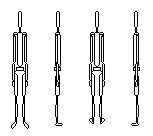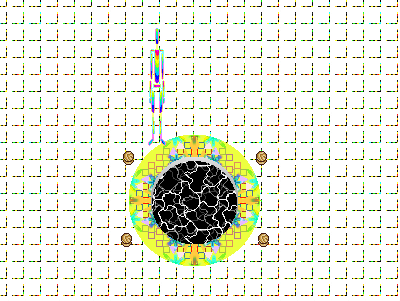Fishing means waiting by the pool with the line in. The longer you wait before pulling the line out, the more likely a creature will appear. Aside from walking, it's the only interaction in the game. The creatures are drawings of things you maybe could find underwater in a dream.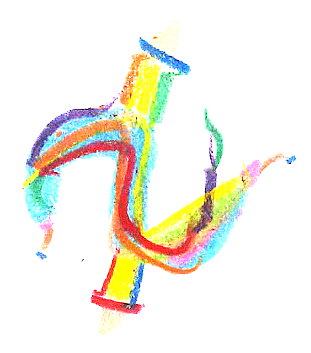The background music is a mix of clips from licensed to share songs on the Free Music Archive. Particularly, Seed64 is an album I used a lot of songs from. The full list of music credits is in the game's README file.

I'm still planning to use the original design in a future version. There would be a reaction-based mini game for catching fish, and the goal would be to catch as many fish as possible within the time limit. I also want to add details and obstacles to the background, which is now a little boring, being a plain, tiled, white floor.

If you want to look at all the drawings or hear the music in the context of the program, there are Windows and source versions available. The source should work on any system with Python and Pygame. If it doesn't, bug reports are much appreciated. Comments are also welcome :)

Dark Stew: Windows, Pygame Source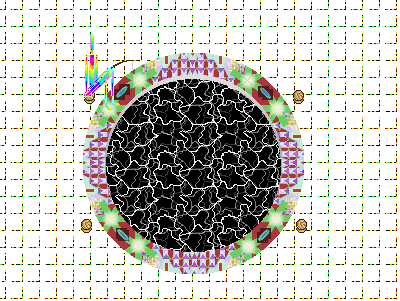I wrote in my last post that I would be working on an old prototype about searching a cloud for organisms for Fishing Jam. I decided to wait a while before developing that game, tentatively titled Xenographic Barrier. Its main interactive element is a first-person scope/flashlight, so I'd like to make a Wii version of it.

I'm about to start working on a complete version of Ball & Cup. If I make anything interesting for it, I'll post something. There are a lot of other things I want to write about, like game analyses, my new GP2X and arcades in Korea, and there's still music to release. Lots of fun stuff coming!